# Ortak Toplayıcı Yükselteç / Common Collector Amplifier

The Common Collector Amplifiers produce an output voltage along the emitter load, which is in the same phase as the input signal. The Common Collector Amplifier is another type of bipolar connection transistor (BJT) in which the input signal is applied to the base terminal and the output signal is received from the emiter terminal. Thus, the collector terminal is common to both input and output circuits. This type of configuration is called the Common Collector (CC), since the collector terminal is effectively "grounded" through the power supply. In many ways, the common collector (CC) configuration is the opposite of the common emitter (CE) configuration, since the connected load resistance is moved from the usual collector terminal labeled RC to the RE labeled emitter terminal. The common collector or grounded collector configuration is usually used when a high impedance input source needs to be connected to a low impedance output load that requires high current gain. Consider the common collector amplifier circuit below.Resistors R1 and R2 create a simple network of voltage dividers used to direct the NPN transistor to transmission. Since this voltage divider slightly loads the transistor, the base voltage can be easily calculated using the simple voltage divider formula, VB, as shown.

## Voltage Divider CircuitWith the collector terminal of the transistor connected directly to the VCC and without collector resistance (RC = 0), any collector current will produce a voltage drop throughout the emitter resistance RE. However, in the common collector amplifier circuit, the same voltage drop, and also the output voltage, represent VOUT. Ideally, we want the DC voltage drop throughout the RE to be equal to half the feed voltage, to ensure that the VCC's silent output voltage of transistors sits somewhere in the middle of its characteristic curves, which allow for a maximum uncut output signal. Therefore, RE selection largely depends on IB and the current gain of transistors beta, β. Since the base-emitter pn-connection is forward-oriented polar, the base current flows from the connection to the emite and promotes transistor movement, causing a much larger collector stream, IC, to flow. Thus, the emitter current is a combination of the basic current and the collector current: IE = IB + IC. However, since the base current is very small compared to the collector current, the emiter current is therefore approximately equal to the collector's current. Thus, as with the IE ≈ IC Common emitter (CE) riser configuration, the input signal is applied to the transistor base terminal and, as we have said before, the output signal of the riser is taken from the emiter emiter terminal. However, since there is only one forward-sided pn-connection between the transistor base and its emitter terminal, any input signal applied to the base passes directly from the connection to the emitter. Therefore, the output signal in the emitter is in the same phase as the input signal applied at the base. Since the output signal of the amplifier is received along the emitter load, this type of transistor configuration is also known as a Transmitter Tracker circuit, except that the emitter output remains about 0.7 volts, as it follows any voltage change in the base input signal. (VBE) below base voltage. Thus, VIN and VOUT are in-phases that produce zero phase differences between input and output signals. Having said this, the absorbers pn-connection effectively acts as an advanced diode and has a resistance to this absorbent diode connection for small AC input signals: r'e = 25mV/Ie is the thermal voltage of the 25mV connection here. room temperature (25oC) and Ie is the emitter current. Thus, as the emitter current increases, the emitter resistance decreases by a proportionate amount. The base current flowing from this built-in base-emitter connection resistance also flows out and passes through the externally connected emitter resistance RE. These two resistors are connected serially, thus acting as a potential dividing network that creates voltage drop. Since the value to r is very small and the RE is usually much larger in the kilohm (kΩ) range, the size of the amplifier's output voltage is therefore less than the input voltage. However, in reality, the size of the output voltage (top to top) is usually worth 98% to 99% of the input voltage and is close enough to be considered a union gain in most cases. Assuming that the basic voltage, VB is actually the input voltage, VIN, we can calculate the voltage gain, VA of the common collector amplifier using the voltage divider formula as shown.

## Common Collector Amplifier Voltage GainTherefore, the common collector amplifier cannot provide voltage amplification, and another expression used to describe the common collector amplifier circuit is the Voltage Tracker Circuit for obvious reasons. Thus, since the output signal closely monitors the input and is in the same phase as the input, the common collector circuit is therefore a unit voltage gain amplifier that does not reverse.

## Common Collector Amplifier Example

A common collector amplifier is created using an NPN bipolar transistor and a voltage divider pre-redeced network. If R1 = 5k6Ω, R2 = 6k8Ω and the supply voltage is 12 volts. When using a load resistance of 4k7Ω: VB, VC and VE, calculate the emitter current IE, internal emitter resistance r and the voltage gain AV values of the riser. Also draw the last circuit and its corresponding characteristic curve with the load line. 1. Base pre-polar stress, VB2. Collector voltage, VC. Since there is no collector load resistance, the transistor collector terminal is connected directly to the DC supply rail, so VC = VCC = 12 volts. 3. Transmitter pre-polarizing voltage, VE4. Transmitter Current, IE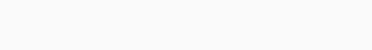5. AC Transmitter Resistance, to r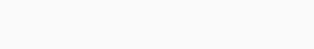6. Voltage gain, AV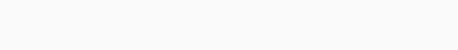## Common Collector Input Impedance

Although the common collector amplifier is not very good at being a voltage amplifier, as we have seen, since the small signal voltage gain is approximately equal to one (AV ≅ 1), however, thanks to it it makes a very good voltage buffer circuit. high input (ZIN) and low output (ZOUT) impedances that provide insulation between an input signal source from a load impedance load. Another useful feature of the common collector amplifier is that it provides current gain (Ai) as long as it is conductive. That is, in response to a small change in the base current, IB, it can pass a large current that flows from the collector to the emitter. Note that this DC stream only sees RE because it is not RC. Then the DC current is simply: VCC/RE, which can be large if RE is small. Consider the following basic common collector riser or transmitter tracker configuration:Capacitors for AC analysis of the circuit short circuit and VCC short circuit (zero impedance). Thus, the equivalent circuit is supplied as shown by the pre-charge currents and voltages given as follows:Input Impedance of the joint collector configuration facing the base, Its ZIN is given as follows:But in Beta, β is usually much larger than 1 (usually over 100), the expression of the following statement: β + 1 can only be reduced to Beta, multiplication with β 100 is almost the same as multiplication with 101.

## Common Collector Amplifier Base Impedance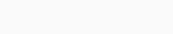Where: β is the current gain of transistors, Re is equivalent emitter resistance and r is ac resistance of the emitter-base diode. Since the combined value of re is usually much larger than the equivalent resistance of diodes, the basic impedance of transistors to r (kilo-ohm compared to a few ohms) can simply be given as follows: β*Re. An interesting point to note here is that the entry base impedance of transistors can be controlled by either the absorbent leg resistance (RE) or the load resistance with the RL value, since they are connected parallel to the ZIN(base). The equation above gives us the input impedance by looking at the base of the transistor, while not the actual input impedance that the source signal will see when it looks at the entire amplifier circuit. For this, we need to take into account the two resistances that make up the voltage dividing bias network. So:

### Common Collector Amplifier Input Impedance## Common Collector Example

Using the previous common collector amplifier circuit above, calculate the input impedances of the transistor base and amplifier stage if the current gain of load resistance, RL 10kΩ and NPN transistors is 100. 1. AC Transmitter Resistance, r to2. Equivalent Load Resistance, Re3. Transistors Base Impedance, ZBASE4. Since the amplifier Input Impedance, ZIN(Stage)322 kΩ transistor base impedance is much higher than that of only 2.8 kΩ amplifier input impedance, the input impedance of the common collector amplifier is determined by the ratio of two front polarization resistances, R1 and R2.

## Common Collector Output Impedance

To determine the output impedance of CC amplifiers ZOUT by looking back at the load riser's emiter terminal, we must lift the load before we want to see the effective resistance of the load-driven riser. Thus, the AC equivalent circuit facing the amplifier output is given as follows:From above, the input impedance of the basic circuit is given as follows:RB = R1|| R2. The current gain of the transistor is given as follows: β. Thus, the output equation is given as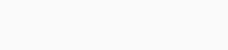follows: Then we can see that the emitter resistance is effectively parallel to the entire impedance of the RE, the transistor facing back to the emitter terminal. If we calculate the output impedance of our common emitter amplifier circuit using the above component values, it gives ZOUT an output impedance lower than the previously calculated ZIN(BASE) high input impedance of 50Ω (49.5Ω). Thus, we can see that the Common Collector amplifier configuration, without calculation, has a very high input impedance and a very low output impedance, which allows it to drive a low impedance load. In fact, due to its relatively high input impedance and very low output impedance due to CC amplifiers, it is widely used as a lucrative buffer amplifier. After the above example determines that the output impedance of the amplifier, ZOUT, is about 50Ω by calculation, now if we reconnect the 10kΩ load resistance to the circuit, the resulting output impedance will be as follows:Although the load resistance is 10kΩ, the equivalent output resistance is still low at 49.3Ω. This is because RL is large compared to ZOUT, so RL should be equal to ZOUT for maximum power transfer. Since the voltage gain of the common collector riser is considered to be union (1), the power gain of the riser must be equal to its current gain in P = V*I. Since the common collector current gain is defined as the ratio of emitter current to base current, γ = IE/IB = β + 1, therefore, it concludes that the current gain of the amplifier should be approximately equal to Beta (β). 1 is almost identical to Beta.

## Summarize

In this tutorial we have seen information about the Common Collector riser, bjt's collector terminal is common in both input and output circuits, since there is no collector resistance, RC. The voltage gain of the common collector riser is approximately equal to the union (Av ≅ 1), and the current gain is approximately equal to Beta, (Ai≅β), depending on the value of certain transistors. We also found through calculation that the input impedance is low in ZOUT, while the output impedance of ZIN is high, and useful for impedance matching (or resistance matching) purposes, or as a buffer circuit between a voltage source and a low impedance load.